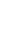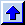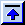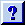# MA2051 - Ordinary Differential EquationsMaple Worksheets - B97

 Worksheet DescriptionIntroductionBrief introduction to dsolve and DEplot. Euler #1Simple blocks of Maple instructions that will do Euler's method for you. Undetermined Coefficients #1A simple block of Maple commands that will do all of the dirty work of undetermined coefficients for you. (A great way to check your answers.) Euler #2This turns the second-order equation into a system and runs Euler's method to build an approximate solution. Have fun! ResonanceThis worksheet generates handles the "general" damped, forced spring-mass system. It allows you to plot the amplitude as a function of the forcing frequency. Limiting BehaviorThis worksheet generates graphs in the phase plane for spring-mass systems. Some of the commands here can be useful when you start work on Project 2. The REAL PendulumThis worksheet generates solution curves for the real, nonlinear pendulum and allows you to compare the results with the linearized model. Competing SpeciesThis worksheet generates solution curves for a population model where two species compete for limited resources. Euler's method (systems)This worksheet implements Euler's method for a nonlinear predator-prey model. Heun's method (systems)This worksheet implements Heun's method for the same predator-prey model. Euler's method (2nd order eqns.)This worksheet implements Euler's method for a damped mass-spring model. Heun's method (2nd order eqns.)This worksheet implements Heun's method for the same mass-spring model.Maintained by: Nathan Gibson, <gibso@wpi.edu>
Last modified: Mon Dec 8, 10:41 EST 1997